Search

About 39 Search Results Matching Types of Worksheet, Worksheet Section, Generator, Generator Section, Type matching Worksheet Section, Grades matching 3rd Grade, Similar to Reading a Protractor Worksheet 2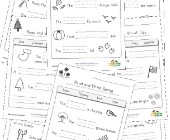Read and Write Worksheets

Choose a word from the word bank to complete each ...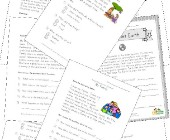This group of free reading worksheets is focussed ...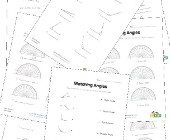Angles Worksheets

Help kids learn about angles with this collection ...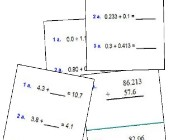Help kids practice decimal addition with the follo...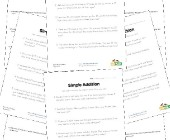Word Problem Worksheets

Help children develop their problem solving skills...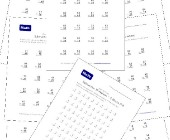Subtraction Worksheets for Kids

This set of free math worksheets is geared towards...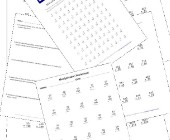Multiplication Worksheets for Kids

This set of math worksheets is geared towards help...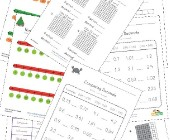Decimal Worksheets

This collection of free decimal worksheets will he...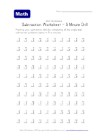Five Minute Subtraction Drill Worksheet

100 simple subtraction problems to do in about 5 m...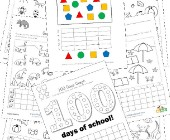Graphing Worksheets

Take a look at our collection of graphing workshee...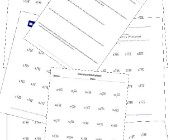Division Worksheets

Help kids learn division with this collection of m...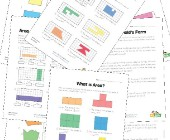Area and Perimeter Worksheets

Help kids learn to calculate the area and perimete...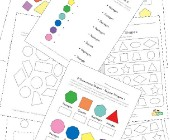Geometry Worksheets

Help kids learn the early basics of geometry inclu...